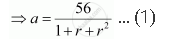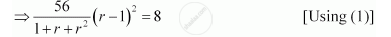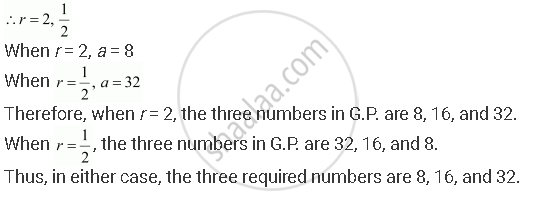CBSE (Arts) Class 11CBSE
Share
Notifications

View all notifications
Books Shortlist
Your shortlist is empty

# The Sum of Three Numbers in G.P. is 56. If We Subtract 1, 7, 21 from These Numbers in that Order, We Obtain an Arithmetic Progression. Find the Numbers. - CBSE (Arts) Class 11 - Mathematics

Login
Create free account

Forgot password?
ConceptRelationship Between A.M. and G.M.

#### Question

The sum of three numbers in G.P. is 56. If we subtract 1, 7, 21 from these numbers in that order, we obtain an arithmetic progression. Find the numbers.

#### Solution

Let the three numbers in G.P. be aar, and ar2.

From the given condition, a + ar + ar2 = 56

⇒ a (1 + r + r2) = 56a – 1, ar – 7, ar2 – 21 forms an A.P.

∴(ar – 7) – (a – 1) = (ar2 – 21) – (ar – 7)

⇒ ar – a – 6 = ar– ar – 14

ar– 2ar + a = 8

ar– ar – ar + a = 8

a(r+ 1 – 2r) = 8

⇒ (r – 1)2 = 8 … (2)⇒7(r2 – 2r + 1) = 1 + r + r2

⇒7r2 – 14 r + 7 – 1 – r – r2 = 0

⇒ 6r2 – 15r + 6 = 0

⇒ 6r2 – 12r – 3r + 6 = 0

⇒ 6r (r – 2) – 3 (r – 2) = 0

⇒ (6r – 3) (r – 2) = 0Is there an error in this question or solution?

#### APPEARS IN

NCERT Solution for Mathematics Textbook for Class 11 (2018 to Current)
Chapter 9: Sequences and Series
Q: 10 | Page no. 199
Solution The Sum of Three Numbers in G.P. is 56. If We Subtract 1, 7, 21 from These Numbers in that Order, We Obtain an Arithmetic Progression. Find the Numbers. Concept: Relationship Between A.M. and G.M..
S